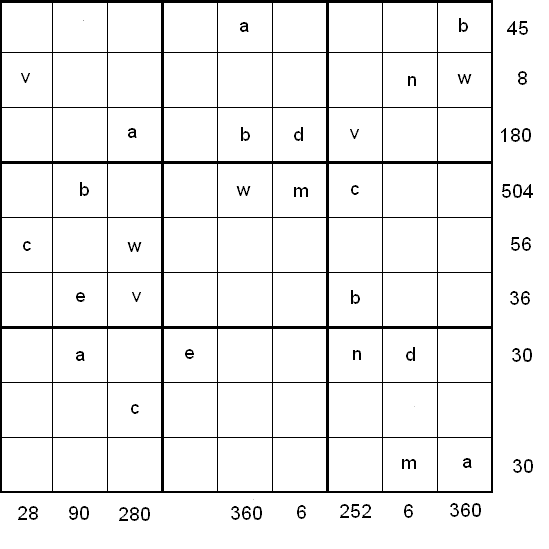#### You may also like### N000ughty Thoughts

How many noughts are at the end of these giant numbers?### DOTS Division

Take any pair of two digit numbers x=ab and y=cd where, without loss of generality, ab > cd . Form two 4 digit numbers r=abcd and s=cdab and calculate: {r^2 - s^2} /{x^2 - y^2}.### Mod 3

Prove that if a^2+b^2 is a multiple of 3 then both a and b are multiples of 3.

# LCM Sudoku

### Why do this problem?

This problem provides an engaging and challenging context in which to practise working with Lowest Common Multiples.

### Possible approach

Show the grid below, or hand out this worksheet."Here is a Sudoku with a difference. As usual:

Each column, each row and each 3x3 box must have the numbers 1 to 9.

No column, row or box can contain the same number more than once.

The nine letters in the grid each represent a different number from 1 to 9."

"What makes this different is that:

The numbers at the end of the rows and at the bottom of the columns are the Lowest Common Multiples (LCM) of the letters in that row.

The LCM of the variables  b, w, m and c in the fourth row is 504."

If necessary, recap with students how to work out LCMs.

"This is a really interesting problem because it can be solved in lots of different ways. Alison, Charlie and Steve worked on this problem. I am going to hand out a record of their work, showing the order in which they worked out the value of the letters. Your challenge is to work out the values of the nine letters in the same order that they did."

Towards the end, bring the class together to compare the merits of different routes.

### Key questions

How many different combinations of three numbers have a LCM of 12?

$180$ appears on row three, and its prime factorisation is $2^2 \times 3^2 \times 5$. What can you deduce for certain about the letters on that row?

### Possible support

Students could work in pairs to make sense of the different routes.

### Possible extension

"Can you work out the values of the letters in any other orders?"